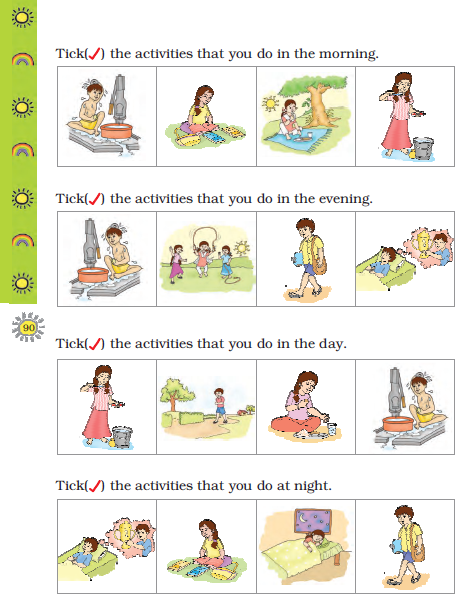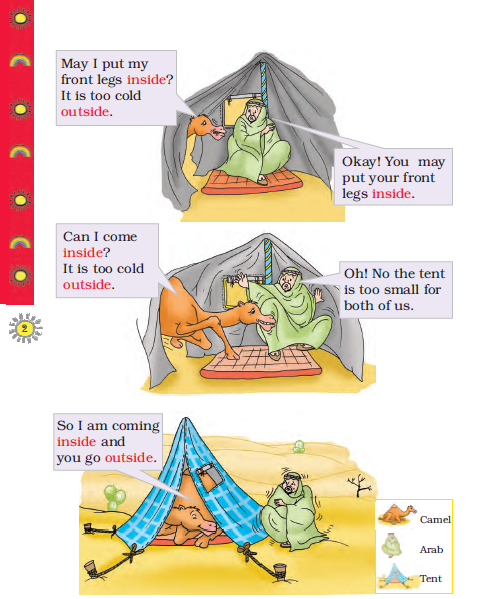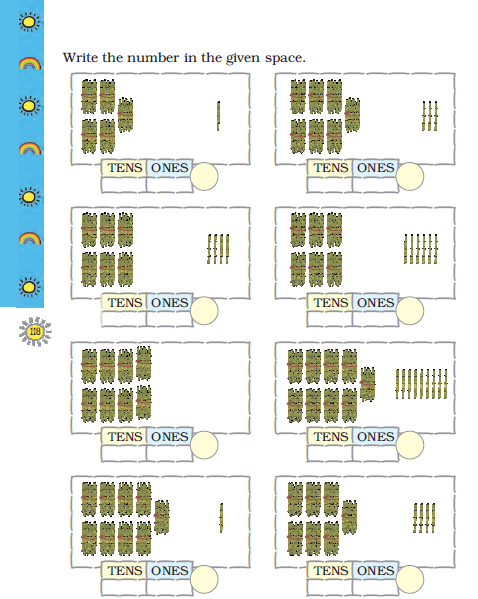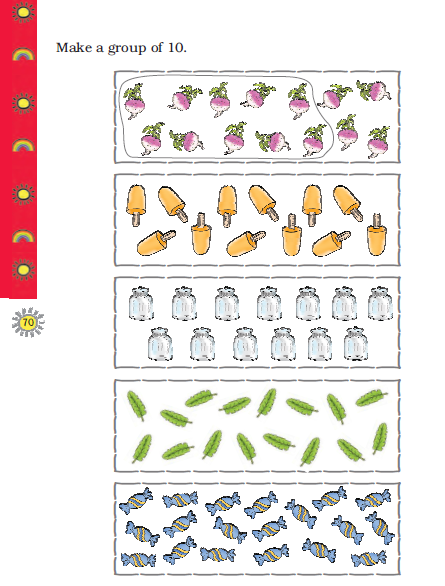CBSE Class 1 Mathematics Books

• NCERT Class 1 Maths Time

Please refer to attached file for NCERT Class 1 Maths - Time

• NCERT Class 1 Maths Subtraction

Please refer to attached file for NCERT Class 1 Maths - Subtraction

• NCERT Class 1 Maths Shapes and Space

Please refer to attached file for NCERT Class 1 Maths - Shapes and Space

• NCERT Class 1 Maths Patterns

Please refer to attached file for NCERT Class 1 Maths - Patterns

• NCERT Class 1 Maths Numbers

Please refer to attached file for NCERT Class 1 Maths - Numbers

NCERT Class 1 Maths TimePlease refer to attached file for NCERT Class 1 Maths - Time

Click for more Mathematics Study Material
 NCERT Class 1 Maths Subtraction NCERT Class 1 Maths Numbers from One to Nine NCERT Class 1 Maths Time NCERT Class 1 Maths Numbers from Ten to Twenty NCERT Class 1 Maths Addition NCERT Class 1 Maths Numbers from Twenty one to Fifty NCERT Class 1 Maths Data Handling NCERT Class 1 Maths Numbers NCERT Class 1 Maths How Many NCERT Class 1 Maths Patterns NCERT Class 1 Maths Measurement NCERT Class 1 Maths Shapes and Space NCERT Class 1 Maths Money

NCERT Class 1 Maths SubtractionPlease refer to attached file for NCERT Class 1 Maths - Subtraction

Click for more Mathematics Study Material
 NCERT Class 1 Maths Patterns NCERT Class 1 Maths Measurement NCERT Class 1 Maths Shapes and Space NCERT Class 1 Maths Money NCERT Class 1 Maths Subtraction NCERT Class 1 Maths Numbers from One to Nine NCERT Class 1 Maths Time NCERT Class 1 Maths Numbers from Ten to Twenty NCERT Class 1 Maths Addition NCERT Class 1 Maths Numbers from Twenty one to Fifty NCERT Class 1 Maths Data Handling NCERT Class 1 Maths Numbers NCERT Class 1 Maths How Many

NCERT Class 1 Maths Shapes and SpacePlease refer to attached file for NCERT Class 1 Maths - Shapes and Space

Click for more Mathematics Study Material
 NCERT Class 1 Maths Time NCERT Class 1 Maths Numbers from Ten to Twenty NCERT Class 1 Maths Addition NCERT Class 1 Maths Numbers from Twenty one to Fifty NCERT Class 1 Maths Data Handling NCERT Class 1 Maths Numbers NCERT Class 1 Maths How Many NCERT Class 1 Maths Patterns NCERT Class 1 Maths Measurement NCERT Class 1 Maths Shapes and Space NCERT Class 1 Maths Money NCERT Class 1 Maths Subtraction NCERT Class 1 Maths Numbers from One to Nine

NCERT Class 1 Maths PatternsPlease refer to attached file for NCERT Class 1 Maths - Patterns

Click for more Mathematics Study Material
 NCERT Class 1 Maths Measurement NCERT Class 1 Maths Shapes and Space NCERT Class 1 Maths Money NCERT Class 1 Maths Subtraction NCERT Class 1 Maths Numbers from One to Nine NCERT Class 1 Maths Time NCERT Class 1 Maths Numbers from Ten to Twenty NCERT Class 1 Maths Addition NCERT Class 1 Maths Numbers from Twenty one to Fifty NCERT Class 1 Maths Data Handling NCERT Class 1 Maths Numbers NCERT Class 1 Maths How Many NCERT Class 1 Maths Patterns

NCERT Class 1 Maths NumbersPlease refer to attached file for NCERT Class 1 Maths - Numbers

Click for more Mathematics Study Material
 NCERT Class 1 Maths Numbers NCERT Class 1 Maths How Many NCERT Class 1 Maths Patterns NCERT Class 1 Maths Measurement NCERT Class 1 Maths Shapes and Space NCERT Class 1 Maths Money NCERT Class 1 Maths Subtraction NCERT Class 1 Maths Numbers from One to Nine NCERT Class 1 Maths Time NCERT Class 1 Maths Numbers from Ten to Twenty NCERT Class 1 Maths Addition NCERT Class 1 Maths Numbers from Twenty one to Fifty NCERT Class 1 Maths Data Handling

NCERT Class 1 Maths Numbers from Twenty one to FiftyPlease refer to attached file for NCERT Class 1 Maths - Numbers from Twenty-one to Fifty

Click for more Mathematics Study Material
 NCERT Class 1 Maths Numbers from One to Nine NCERT Class 1 Maths Time NCERT Class 1 Maths Numbers from Ten to Twenty NCERT Class 1 Maths Addition NCERT Class 1 Maths Numbers from Twenty one to Fifty NCERT Class 1 Maths Data Handling NCERT Class 1 Maths Numbers NCERT Class 1 Maths How Many NCERT Class 1 Maths Patterns NCERT Class 1 Maths Measurement NCERT Class 1 Maths Shapes and Space NCERT Class 1 Maths Money NCERT Class 1 Maths Subtraction

NCERT Class 1 Maths Numbers from Ten to TwentyPlease refer to attached file for NCERT Class 1 Maths - Numbers from Ten to Twenty

Click for more Mathematics Study Material
 NCERT Class 1 Maths Numbers NCERT Class 1 Maths How Many NCERT Class 1 Maths Patterns NCERT Class 1 Maths Measurement NCERT Class 1 Maths Shapes and Space NCERT Class 1 Maths Money NCERT Class 1 Maths Subtraction NCERT Class 1 Maths Numbers from One to Nine NCERT Class 1 Maths Time NCERT Class 1 Maths Numbers from Ten to Twenty NCERT Class 1 Maths Addition NCERT Class 1 Maths Numbers from Twenty one to Fifty NCERT Class 1 Maths Data Handling

NCERT Class 1 Maths Numbers from One to NinePlease refer to attached file for NCERT Class 1 Maths - Numbers from One to Nine

Click for more Mathematics Study Material
 NCERT Class 1 Maths Addition NCERT Class 1 Maths Numbers from Twenty one to Fifty NCERT Class 1 Maths Data Handling NCERT Class 1 Maths Numbers NCERT Class 1 Maths How Many NCERT Class 1 Maths Patterns NCERT Class 1 Maths Measurement NCERT Class 1 Maths Shapes and Space NCERT Class 1 Maths Money NCERT Class 1 Maths Subtraction NCERT Class 1 Maths Numbers from One to Nine NCERT Class 1 Maths Time NCERT Class 1 Maths Numbers from Ten to Twenty

NCERT Class 1 Maths MoneyPlease refer to attached file for NCERT Class 1 Maths - Money

Click for more Mathematics Study Material
 NCERT Class 1 Maths Numbers from Twenty one to Fifty NCERT Class 1 Maths Data Handling NCERT Class 1 Maths Numbers NCERT Class 1 Maths How Many NCERT Class 1 Maths Patterns NCERT Class 1 Maths Measurement NCERT Class 1 Maths Shapes and Space NCERT Class 1 Maths Money NCERT Class 1 Maths Subtraction NCERT Class 1 Maths Numbers from One to Nine NCERT Class 1 Maths Time NCERT Class 1 Maths Numbers from Ten to Twenty NCERT Class 1 Maths Addition

Latest NCERT & CBSE News

Read the latest news and announcements from NCERT and CBSE below. Important updates relating to your studies which will help you to keep yourself updated with latest happenings in school level education. Keep yourself updated with all latest news and also read articles from teachers which will help you to improve your studies, increase motivation level and promote faster learning

This is with reference to CBSE Notification No. 40/2021 dated 04.05.2021 regarding the Innovation Ambassador program – An online training program for teachers by CBSE in collaboration with Ministry of Education’s Innovation Cell (MIC) and AICTE. In view of the current...

MCQ Question based CBSE examination

For 2021-22 CBSE has launched MCQ question-based examination for Term 1 & Term 2 board examinations. The entire syllabus has been divided into two parts each including 50% of the entire syllabus. To score well, students must practice as per the new CBSE term-wise...

All India Children Educational Audio Video Festival

The Central Institute of Educational Technology (CIET), a constituent unit of National Council of Educational Research and Training (NCERT), is inviting entries for the 26th All India Children’s Educational Audio Video Festival (AICEAVF). This festival showcases the...

How To Solve Unseen Passages In English

Unseen passages may contain one or many paragraphs. This is one of the important yet easy parts for a student to get marks. Students should thoroughly study and understand the passage to answer the related questions. The unseen passages are there just to test the...

CBSE Class 10 Revised Syllabus

Last year CBSE had to reduce the syllabus because of the pandemic situation but it was not very effective because there were no examinations. This year to avoid any confusion and conflict, CBSE has decided to reduce the syllabus into term 1 and term 2. 50 percent of...

CBSE OMR Sheet Guidelines for Term 1

You are aware that the CBSE will be using OMR for the first time for assessment of both the Classes-X and XII in Term-I examinations. Hence, there is a need that all the students appearing in the Term-l examinations and the schools sponsoring these students should have...